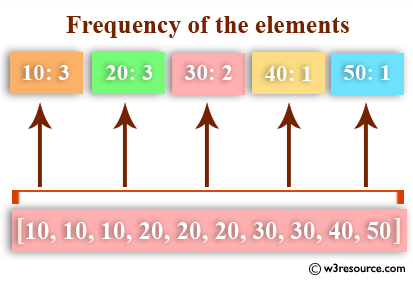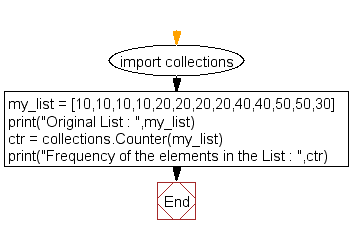﻿ Python: Get the frequency of the elements in a list - w3resource# Python: Get the frequency of the elements in a list

## Python List: Exercise - 30 with Solution

Write a Python program to get the frequency of the elements in a list.Sample Solution:-

Python Code:

``````import collections
my_list = [10,10,10,10,20,20,20,20,40,40,50,50,30]
print("Original List : ",my_list)
ctr = collections.Counter(my_list)
print("Frequency of the elements in the List : ",ctr)
```
```

Sample Output:

```Original List :  [10, 10, 10, 10, 20, 20, 20, 20, 40, 40, 50, 50, 30]
Frequency of the elements in the List :  Counter({10: 4, 20: 4, 40: 2, 50: 2, 30: 1})
```

Flowchart:## Visualize Python code execution:

The following tool visualize what the computer is doing step-by-step as it executes the said program:

Python Code Editor:

Have another way to solve this solution? Contribute your code (and comments) through Disqus.

What is the difficulty level of this exercise?

Test your Python skills with w3resource's quiz

﻿

## Python: Tips of the Day

Floor Division:

When we speak of division we normally mean (/) float division operator, this will give a precise result in float format with decimals.

For a rounded integer result there is (//) floor division operator in Python. Floor division will only give integer results that are round numbers.

```print(1000 // 300)
print(1000 / 300)```

Output:

```3
3.3333333333333335```